# Forms of Energy energy kinetic energy potential energy

• Slides: 17Forms of Energy • • • energy kinetic energy potential energy work mechanical energy • • • sound energy thermal energy electric energy radiant energy nuclear energy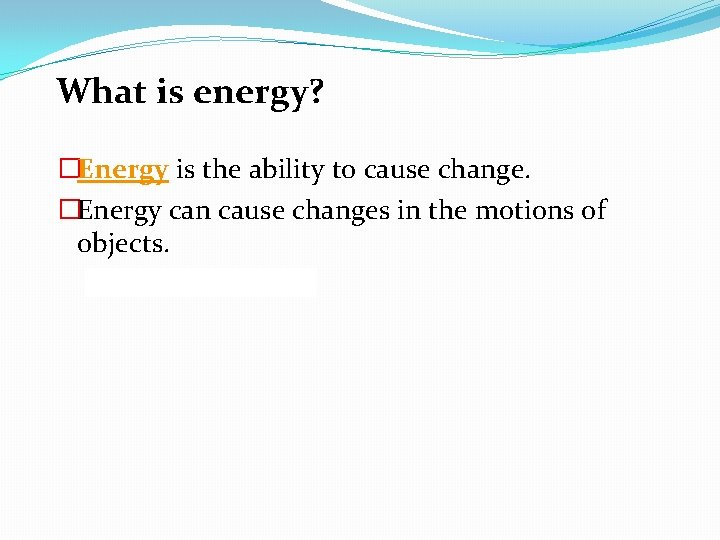What is energy? �Energy is the ability to cause change. �Energy can cause changes in the motions of objects.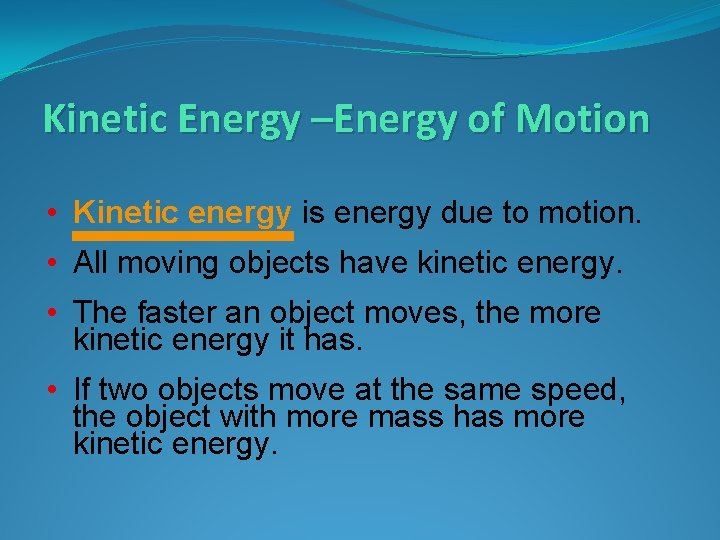Kinetic Energy –Energy of Motion • Kinetic energy is energy due to motion. • All moving objects have kinetic energy. • The faster an object moves, the more kinetic energy it has. • If two objects move at the same speed, the object with more mass has more kinetic energy.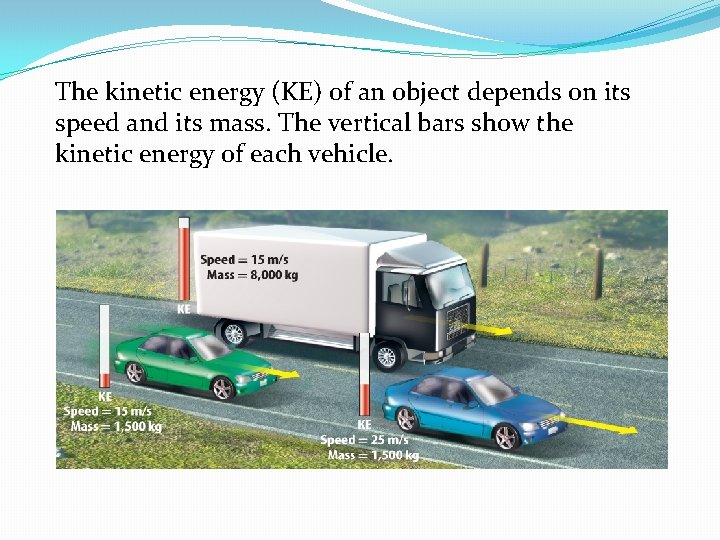The kinetic energy (KE) of an object depends on its speed and its mass. The vertical bars show the kinetic energy of each vehicle.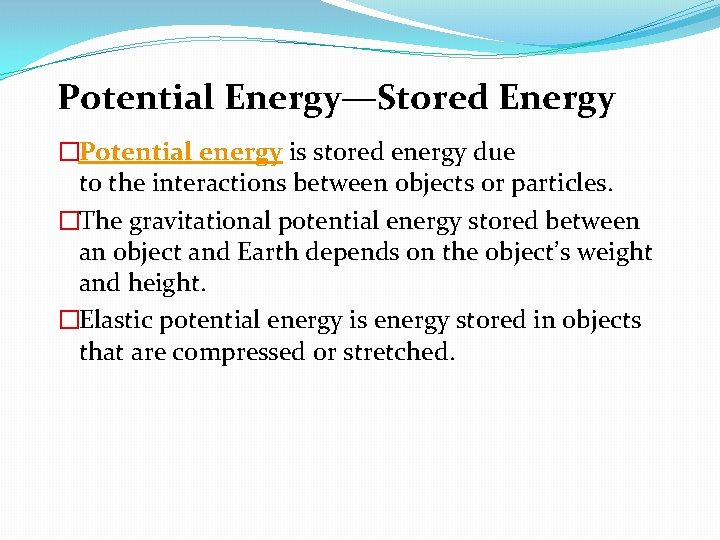Potential Energy—Stored Energy �Potential energy is stored energy due to the interactions between objects or particles. �The gravitational potential energy stored between an object and Earth depends on the object’s weight and height. �Elastic potential energy is energy stored in objects that are compressed or stretched.Chemical potential energy is energy stored in the chemical bonds between atoms.Energy and Work �Work is the transfer of energy that occurs when a force is applied over a distance. �Work depends on both force and distance. �You only do work on an object if that object moves. �All energy can be measured in joules (J).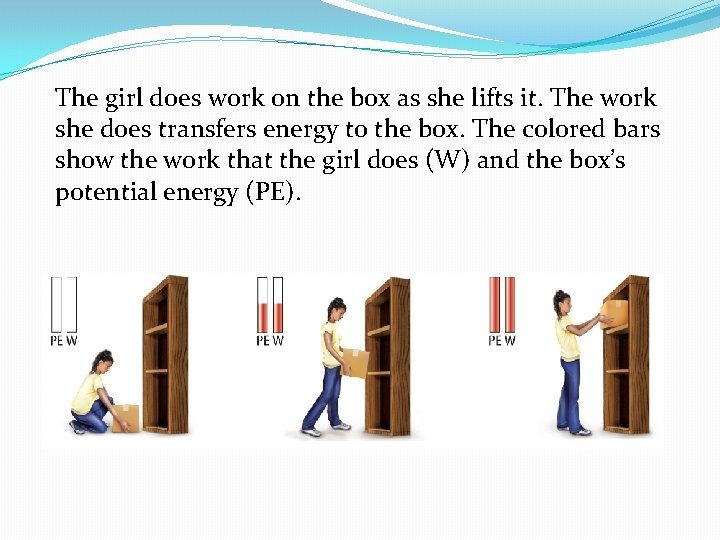The girl does work on the box as she lifts it. The work she does transfers energy to the box. The colored bars show the work that the girl does (W) and the box’s potential energy (PE).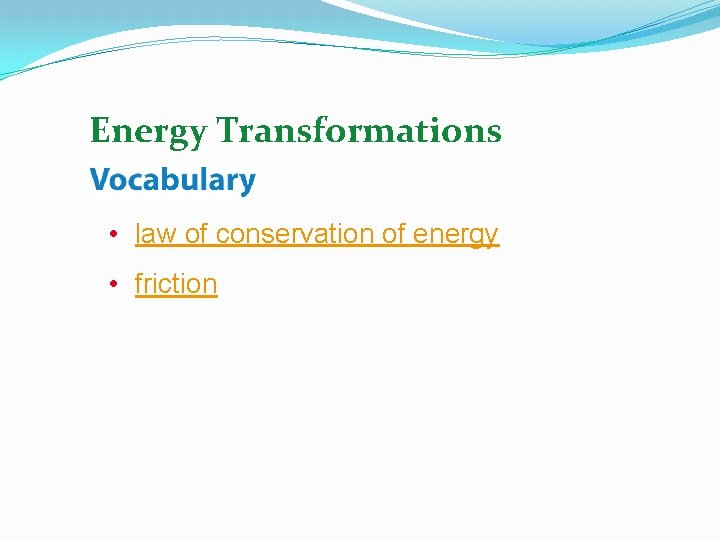Energy Transformations • law of conservation of energy • friction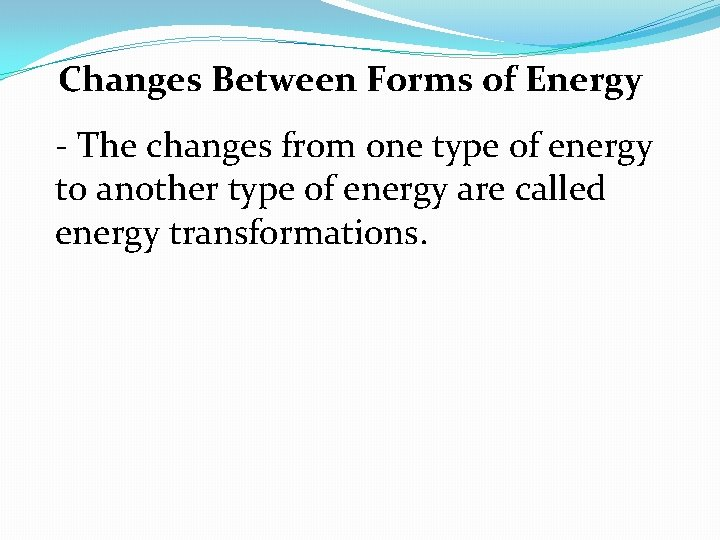Changes Between Forms of Energy - The changes from one type of energy to another type of energy are called energy transformations.A microwave oven changes electric energy into radiant energy.Changes Between Kinetic and Potential Energy changes between kinetic energy (KE) and potential energy (PE) when a ball is thrown and moves upward and then downward.�As the ball moves upward, its speed and kinetic energy decrease, but the potential energy is increasing because the ball’s height is increasing. �At the ball’s highest point, the gravitational potential energy is greatest, and the ball’s kinetic energy is the least.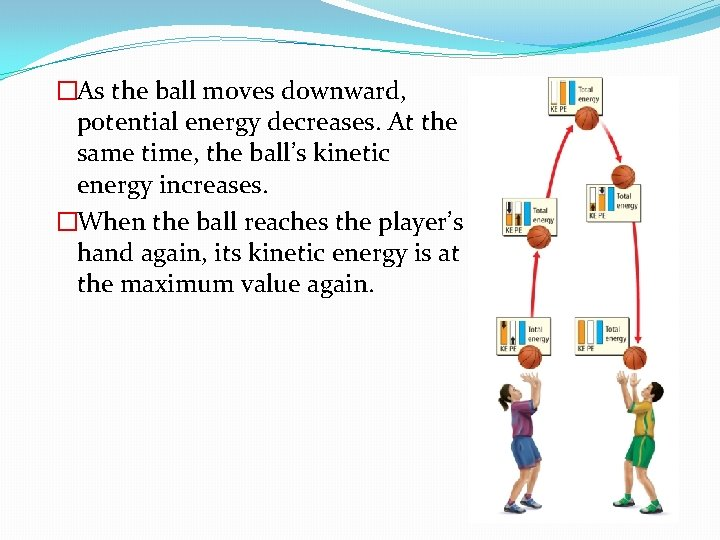�As the ball moves downward, potential energy decreases. At the same time, the ball’s kinetic energy increases. �When the ball reaches the player’s hand again, its kinetic energy is at the maximum value again.The Law of Conservation of Energy According to the law of conservation of energy, energy can be transformed from one form into another or transferred from one region to another, but energy cannot be created or destroyed. Friction is a force that resists the sliding of two surfaces that are touching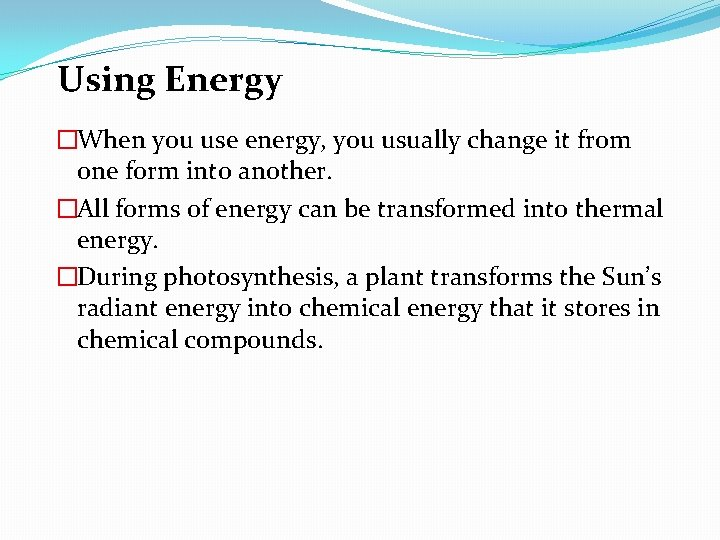Using Energy �When you use energy, you usually change it from one form into another. �All forms of energy can be transformed into thermal energy. �During photosynthesis, a plant transforms the Sun’s radiant energy into chemical energy that it stores in chemical compounds.Using Energy (cont. ) �When energy changes form, some thermal energy is always released. �Scientists often refer to thermal energy that cannot be used as waste energy. �Whenever energy is used, some energy is transformed into useful energy and some is transformed into waste energy.